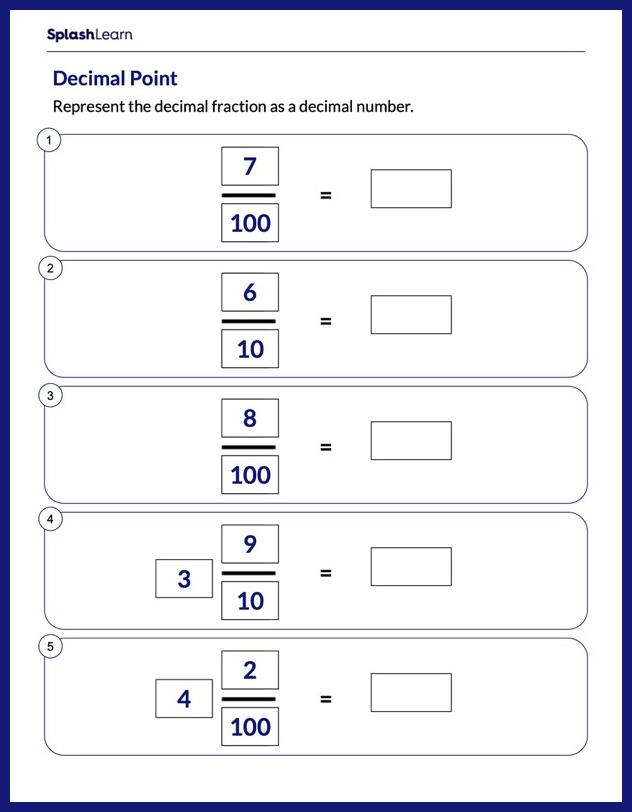# Represent Decimal Fractions as Decimal Number Worksheet

Home > Represent Decimal Fractions as Decimal NumberStruggles with converting to decimals can easily be overcome if students practice the concept in a fun and engaging way! The worksheet challenges young mathematicians to hone their math skills by solving a set of problems on representing decimal fractions to decimal numbers. Regular practice will help your students develop confidence in the classroom and in the real world.